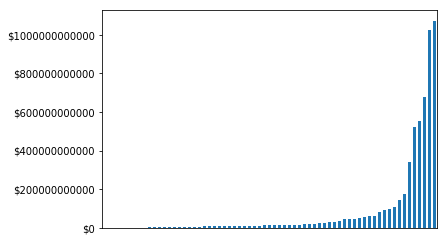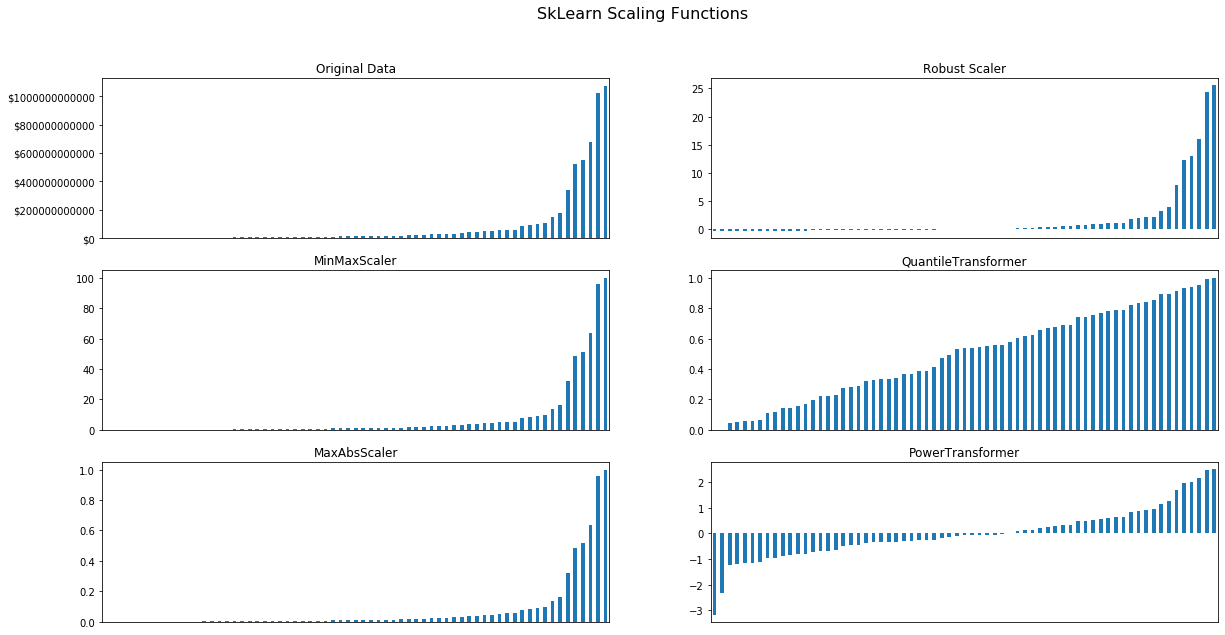Scaling Data

Scaling data is a constant challenge in analytics projects. Whether to get a visualization to fit on screen or to take a independent variable and remove outliers scaling transforms data from working in one domain or another.

Let's think about this by example, let's say you wanted to analyze the US Government budget. You would find that the US Federal government spends about \$2 Billion on deposit insurance and \\$1 Trillion on Medicare. A huge range. But what if we wanted to know about what share that would be if the US National budget were only \$100. In that case we would scale the budgets to between \$0.00 and \\$100. There are many approaches and mathematical ways to scale the data. In this cookbook, we will compare all of the approaches in an attempt to pick the best one. About the data¶ The dataset for this analysis is the US Budget Federal Account balances which can be downloaded from US Spending website. let's load the data and take a look. Specifically, we are going to focus in on three main columns: • budget_authority_appropriated_amount: The amount congress decided this account should have. In : import pandas as pd import matplotlib.pyplot as plt pd.options.display.float_format = '${:,.2f}'.format

Out:
federal_account_symbol federal_account_name agency_name budget_function budget_subfunction budget_authority_unobligated_balance_brought_forward adjustments_to_unobligated_balance_brought_forward budget_authority_appropriated_amount borrowing_authority_amount contract_authority_amount spending_authority_from_offsetting_collections_amount total_other_budgetary_resources_amount total_budgetary_resources obligations_incurred deobligations_or_recoveries_or_refunds_from_prior_year unobligated_balance gross_outlay_amount status_of_budgetary_resources_total
0 070-5702 9-11 Response and Biometric Exit Account, U.S.... Department of Homeland Security Administration of Justice Federal law enforcement activities $65,767,068.87$4,895,455.21 $59,911,887.98$0.00 $0.00$0.00 $0.00$130,574,412.06 $81,302,583.39$4,895,455.21 $49,271,828.67$69,493,561.19 $130,574,412.06 1 014-5015 Abandoned Mine Reclamation Fund, Office of Sur... Department of the Interior Natural Resources and Environment Conservation and land management$173,858,680.72 $21,873,656.93$379,980,611.05 $0.00$0.00 $952.77$952.77 $575,713,901.47$402,411,947.53 $21,873,656.93$173,301,953.94 $285,538,947.66$575,713,901.47
2 014-2640 Abandoned Well Remediation Fund, Bureau of Lan... Department of the Interior Natural Resources and Environment Conservation and land management $447,121.09$5.27 $0.00$0.00 $0.00$0.00 $0.00$447,126.36 $285,815.33$5.27 $161,311.03$9,415,726.69 $447,126.36 3 068-0118 Abatement, Control and Compliance Loan Program... Environmental Protection Agency Natural Resources and Environment Pollution control and abatement$0.46 $-0.39$0.00 $0.00$0.00 $0.00$0.00 $0.07$0.00 $0.00$0.07 $0.00$0.07
4 069-0503 Access Highways to Public Recreation Areas on ... Department of Transportation Transportation Ground transportation $352,333.19$0.00 $0.00$0.00 $0.00$0.00 $0.00$352,333.19 $0.00$0.00 $352,333.19$0.00 $352,333.19 Let's group the data by the "budget subfunction" as defined by the Department of the treasury. In : budget_function_data = budget_data_raw.groupby(["budget_subfunction"]).sum()['budget_authority_appropriated_amount'].sort_values() budget_function_data.plot(kind='bar') ax1 = plt.axes() x_axis = ax1.axes.get_xaxis().set_visible(False) ax1.yaxis.set_major_formatter(FormatStrFormatter('$%.0f'))Sci-Kit Learn contains most of the libraries necessary to support scaling in a wide variety of use cases.

Below we will create a pipeline that will call five of the most common scaling functions.

In :
from sklearn.preprocessing import *
from sklearn import pipeline
from sklearn_pandas import DataFrameMapper

mapper = DataFrameMapper([
(['budget_authority_appropriated_amount'], MinMaxScaler(feature_range=(0,100))
, {'alias': 'MinMaxScaler'}),
(['budget_authority_appropriated_amount'], MaxAbsScaler()
, {'alias': 'MaxAbsScaler'}),
(['budget_authority_appropriated_amount'], RobustScaler()
, {'alias': 'RobustScaler'}),
(['budget_authority_appropriated_amount'], QuantileTransformer(n_quantiles=10)
, {'alias': 'QuantileTransformer'}),
(['budget_authority_appropriated_amount'], PowerTransformer()
, {'alias': 'PowerTransformer'})
], df_out=True)

scaled_data = mapper.fit_transform(budget_function_data.reset_index())

and let's take a look at the results:

In :
import matplotlib.pyplot as plt
from matplotlib.ticker import ScalarFormatter, FormatStrFormatter

fig = plt.figure(figsize=(20,10))
fig.suptitle('SkLearn Scaling Functions', fontsize=16)
# 3 x 1 grid, position at (1st row, 1st col), take two rows

ax0 = plt.subplot2grid((3, 2), (0, 0), rowspan=1)

budget_function_data.plot(kind='bar', title='Original Data')
ax0.yaxis.set_major_formatter(FormatStrFormatter('$%.0f')) ax1 = plt.subplot2grid((3, 2), (1, 0), rowspan=1) scaled_data['MinMaxScaler'].plot(kind='bar', title='MinMaxScaler') ax2 = plt.subplot2grid((3, 2), (2, 0), rowspan=1) scaled_data['MaxAbsScaler'].plot(kind='bar', title='MaxAbsScaler') ax3 = plt.subplot2grid((3, 2), (0, 1), rowspan=1) scaled_data['RobustScaler'].plot(kind='bar', title='Robust Scaler') ax4 = plt.subplot2grid((3, 2), (1, 1), rowspan=1) scaled_data['QuantileTransformer'].plot(kind='bar', title='QuantileTransformer') # get the other ax ax5 = plt.subplot2grid((3, 2), (2, 1), rowspan=1) scaled_data['PowerTransformer'].plot(kind='bar', title='PowerTransformer') for axis in fig.get_axes(): axis.get_xaxis().set_visible(False)The effects and drawbacks should be fairly obvious. Both MinMaxScaler and MaxAbsScaler keep the same overall distribution. The relative difference between say "Executive Management" and "Medicare", which have budgets that are about 2000x different, are maintained. RobustScaler appears the same but it subtly different. It is based on centering between the 75th and 25th percentiles. In this case Medicare and some of the smaller accounts are effectively clipped at the 25th and 75th percentile. Thus, outliers are managed the the relative differences are maintained for the whole population. The last two PowerTransformer and QuantileTransformer are deliberately manipulative. Each force the population to fit a specific distribution. This removes the vast differences in scale inherent in the data and makes things seem more comparable. But beware, looking at these charts it appears that Medicare and the Defense department are just a little bit more expensive than say interest payments on debt; but, they are an order of magnitude different as shown below: In : budget_function_data.tail() Out: budget_subfunction Interest on Treasury debt securities (gross)$521,553,461,024.26
Health care services                             $551,865,968,531.50 Department of Defense-Military$679,616,519,190.72
Social security                                $1,025,474,293,425.75 Medicare$1,072,980,747,544.25
Name: budget_authority_appropriated_amount, dtype: float64
In [ ]: